# Split Half Method: Definition, Examples

The parallel-forms method, in many instances, is expensive and frequently difficult to construct. Therefore, a less direct method of assessing the effects of different samples of items is the so-called split-half method. Only one test is administered to compute the reliability coefficient in this technique.

The whole set of test-items is divided into two equal halves. Then a test can be administered, and separate scores assigned to every individual on two arbitrarily selected halves of that test can be obtained.

For example, an individual may be given one score on the odd-numbered items and a second score on the even-numbered items.

Then the product­ moment correlation between the two sets of scores gives the parallel-forms reliability coefficient for a test half as long as the original test.

A notable problem arises in the split-half technique while splitting the test in order to obtain the most nearly comparable halves.

In most tests, the first half and the second half would not be comparable owing to differences in nature and difficulty level of items.

A procedure that is adequate for most purposes is to find the scores on odd and even-numbered items of the test. If the items were originally arranged in approximate order of difficulty, such a division yields very nearly equivalent half­scores.

Once the two-half scores have been obtained for each individual, they may be correlated by the usual method. It should be noted, however, that correlation actually gives the reliability of only a half test.

For example, if the entire test consists of 100 items, the correlation is computed between sets of scores, each of which is based on only 50 items.

In both test-retest and parallel-forms reliability, on the other hand, each score is based on the full number of items in the test.

Assuming that the two halves are equivalent, the reliability of the full test ( rtt ) can be estimated by means of the Spearman-Brown prophecy formula, as given below.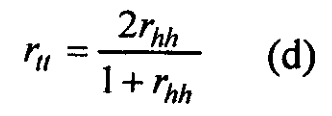Where rhh is the correlation between the half test. As an example, if the correlation of the total scores on the odd-numbered items with total scores of the even-numbered items is 0.80, the estimated reliability of the whole test is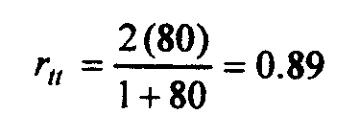The Spearman-Brown prophecy formula stated in (d) above is a particular case of a more general formula of the following type:in which k is the factor by which the test is to be lengthened or shortened with respect to the original test whose reliability coefficient is ro.

The formula (e) is of particular significance. It gives an estimate of the effect of lengthening or shortening a test on its reliability coefficient. Suppose we have an n1 item test, and we know its reliability, which is r0.

By the use of this formula, we can predict what its reliability would be if n2 additional similar items were added to the test. Here k = ( n1 + n2 ) / n1 . Substituting k in (e), the reliability coefficient of the new test can be calculated.

Similarly, if we have an m1 item test of known reliability and we wish to reduce it to a test of m2 item (m2 < m1), Spearman-Brown formula may be employed with k = ( m1 – m2 )  to estimate the reliability of the shortened test.

In addition, the formula is useful when we want to determine how many items will be needed to achieve a given level of reliability.

For instance, if rtt were set at 0.9, we can determine how many items would be needed inter-correlating 0.5 to achieve this desired level of reliability. This can be obtained from the following formula.The formula can easily be obtained from a sample rearrangement of (e). Setting rtt=0.90, and ro=O.5O, we find;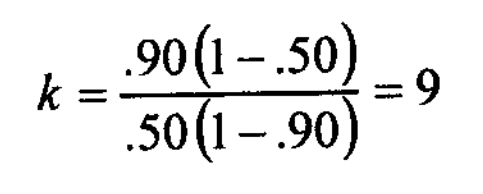This demonstrates the main justification for including a number of items in a test (or scale) that the reliability can thereby be increased to a satisfactory level.

The formula further shows that the number of items needed to reach a given level of reliability depends on the homogeneity of the items that are on the inter-correlations between them.

Example #1

Suppose we have a 20-item test with a reliability coefficient

0.60. Estimate what the reliability of this test would be if 80 similar items were added to make it a 100-item test.

Solution: In this instance k = ( n1 + n2 ) / n1 = (20 + 80 ) / 20 = 5 and r0 = 0,60.

Hence using (e);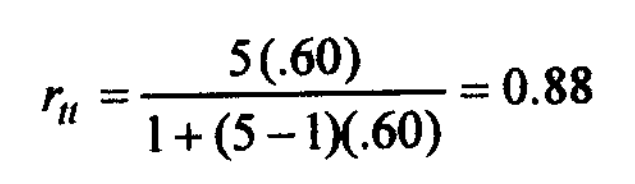Example #2

Suppose we have a 110-item test, the length of which is reduced to 55 items. The reliability coefficient of the original test is 0.80. What would be the reliability of the shortened test?

Solution: Here k = ( m1 – m2 ) / m1 = ( 110 – 55 ) 110 = 0.50 and r0 = 0.80.

Hence;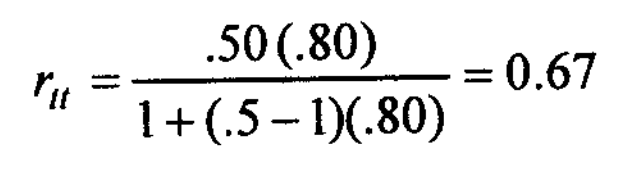An alternative method for finding split-half reliability was developed by Rulon (1939).

It requires only the variance of the differences between each person’s scores and the two half-tests ( s2e ) and the variance of total scores ( s2t ); these two values are substituted in the following formula, which yields the reliability of the whole test directly:Thus for a test with a standard deviation of 6 and a standard error of measurement 3, the Rulon’s method gives a reliability coefficient of;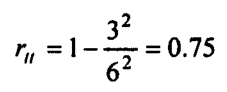Example #3

A sample of ten students, all of the same age, was selected and given a Math test to assess strengths and weaknesses in a number of math and math-related areas.

Use the split-half method with a Spearman-Brown correction for the desired assessment. The scaled scores for the odd and even items are shown in the accompanying table.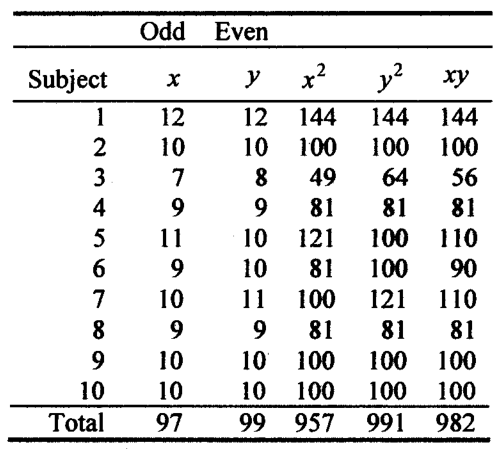The computed correlation coefficient is;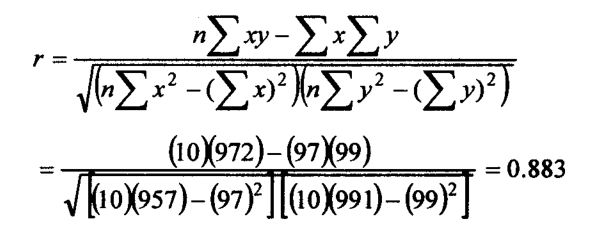With 8 df, the r is significant at a 1% level (for r to be significant, a table value of 0.765 is required). Since r is significant, we apply the Spearman-Brown formula as follows: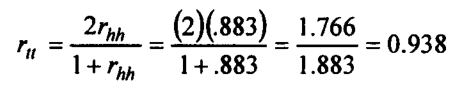The reliability is thus established at 0.938, which is certainly a lofty value and indicates a high degree of reliability.

More 'Reliability and Validity in Measurement' Posts ⁄
Related Posts ⁄